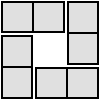#### You may also like### 4 Dom

Use these four dominoes to make a square that has the same number of dots on each side.### Pair Sums

Five numbers added together in pairs produce: 0, 2, 4, 4, 6, 8, 9, 11, 13, 15 What are the five numbers?### Number Sandwiches

Can you arrange the digits 1, 1, 2, 2, 3 and 3 to make a Number Sandwich?

# One to Eight

##### Age 11 to 14Challenge Level

Here are some 'funny factorisations'. Complete the following expressions so that each one gives a four digit number as the product of two two digit numbers and uses the digits $2$ to $9$ once and only once.

** $\times$ ** $= 4876$
** $\times$ ** $= 5394$

Now do the same with the digits $1$ to $8$ to complete the following expressions.

** $\times$ ** $= 1368$
$5$* $\times$ $6$* $=$ ****
$52$ $\times$ ** $=$ ****

Is there more than one solution?